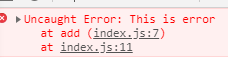# ES6箭头函数和扩展实例分析

更新时间：2020年05月23日 11:07:51   转载 作者：WFaceBoss1.默认值

```function add(a,b=1){
return a+b;
}

2.主动抛出错误

ES6中我们直接用throw new Error( xxxx ),就可以抛出错误。

```function add(a,b=1){
if(a == 0){
throw new Error('This is error')
}
return a+b;
}3.函数中的严谨模式

```function add(a,b=1){
'use strict'
if(a == 0){
throw new Error('This is error');
}
return a+b;
}

```function add(a,b){
'use strict'
if(a == 0){
throw new Error('This is error');
}
return a+b;
}

4.获得需要传递的参数个数

ES6为我们提供了得到参数的方法(xxx.length).我们用上边的代码看一下需要传递的参数个数。

```function add(a,b){
'use strict'
if(a == 0){
throw new Error('This is error');
}
return a+b;
}

```function add(a,b=1){

if(a == 0){
throw new Error('This is error');
}
return a+b;
}

5.箭头函数

```var add =(a,b=1) => {
console.log('hello world')
return a+b;
};